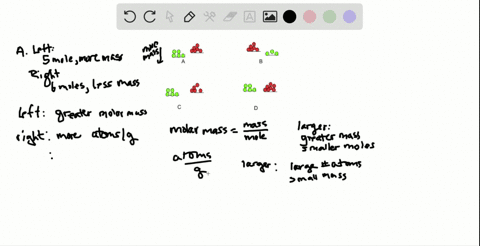🎉 The Study-to-Win Winning Ticket number has been announced! Go to your Tickets dashboard to see if you won! 🎉View Winning Ticket### Each of the following balances weighs the indicat…

11:00University of Maine
Problem 6

# You need to calculate the number of $\mathrm{P}_{4}$ molecules that can form from 2.5 $\mathrm{g}$ of $\mathrm{Ca}_{3}\left(\mathrm{PO}_{4}\right)_{2} .$ Draw a road map for solving this and write a Plan, without doing any calculations.

## Discussion

You must be signed in to discuss.

## Video Transcript

in a chemical reaction. You can go from one substance to another using the balanced equation. So the balanced equation describes the ratio of substances in terms of moles. So your first step is toe always right. The balanced equation. Using this, you can convert from one substance to another, looking at things in terms of grams, moles, molecules or atoms. So if you know that you have a certain quantity in mass or grams of an initial substance calcium phosphate, we can use our information what we know about relationships between mass and moles as well as the balanced equation, to find out how many molecules of a product which in this case is P four. So I have an initial substance reacted and a product without writing the balanced equation for this. I know that initially I have grams of see a three p a. For to. The first step is to change that two moles cause their balanced equation always deals with moules, and we do this by dividing by the molar mass of calcium phosphate. Once we have moles of calcium phosphate, we can switch two malls of a new substance. In this case, p four and we do that using the mole ratio from the balanced equation. So we multiply by the moles of P for over the moles of See a three p o for to, and this is called the mole ratio, and it's found in the balanced equation. Once we have moles of P four, we can convert it to molecules because we know the relationship between moles and molecules uses of a God rose number. So we'll multiply by of a God rose number or six 102 times 10 to the 23rd. So these steps allow you to go from one substance to another, and by knowing the relationships between things, we confined different quantities.